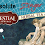## Wednesday, August 26, 2015

### Biased Estimation of Marginal Effects

I began a recent post with the comment:
"One thing that a lot of practitioners seem to be unaware of (or they choose to ignore it) is that in many of the common situations where we use regression analysis to estimate elasticities, these estimators are biased.
And that's true even if all of the conditions needed for the coefficient estimator (e.g., OLS) to be unbiased are fully satisfied."
Exactly the same point can be made in respect of estimated marginal effects, and that's what this post is about.

As in the earlier post, we'll consider four simple regression models (and once again, although we're dealing with models that have a single regressor, all of the results below still apply in the multiple regression case.):

LIN-LIN:       yi = α + β xi + εi

LOG-LOG:  log(yi) = α + β log(xi) + εi

LIN-LOG:  yi = α + β log(xi) + εi

LOG-LIN:   log(yi) = α + β xi + εi

In each case, we'll assume x is non-random and that the εi's are i.i.d. [0 , σ2]; i = 1, 2, ..., n. "Log" denotes "natural logarithm". (Note that normality of the errors is not being assumed.)

Now, let's consider the marginal effect of a change in x on y.

The LIN-LIN and LIN-LOG models are easily dispensed with. In the former case the marginal effect is just the parameter, β. In the LIN-LOG model it is (β / xi). So, if we have an unbiased estimator of β (such as OLS) we immediately have an unbiased estimator of the marginal effect. (Recall that the regressor is non-random.)

The situation is more complicated for the LOG-LOG and LOG-LIN models. For the LOG-LOG model, the marginal effect at observation i is (β xi / yi), where yi is random. So, even if we estimate beta with an unbiased estimator, such as OLS, the corresponding estimator of the marginal effect will be biased. This is essentially because the latter estimator is a non-linear function of the random data.

In the case of the LOG-LIN model, the marginal effect is (β / yi), and the same general point applies when it comes to the properties of the usual estimator of this marginal effect.

If we estimate the marginal effect at the sample mean(s) of the data, a similar problem arises. The values of the biases alter in detail, but that's all.

In a recent paper, I evaluate the expressions for these biases, obtaining analytical expressions using a "small-disturbance" ("small-sigma") approximation.

Not surprisingly, these expressions are quite messy, but the directions of the biases can be signed in some cases. I also provide some numerical evaluations. The latter show that the magnitudes of the biases can be considerably larger than those associated with elasticity estimators. I also find that the results are very robust to the form of the distribution for the error term in the underlying regression models.

Reference

Giles, D. E., 2015. The biases of marginal effect estimators in log-transformed regression models. Mimeo., Department of Economics, University of Victoria.

1.1.